# Preface

This section is devoted to an important class of nonlinear system of equations---gradient systems. The reason why gradient systems are grouped together is that for such systems there is a natural candidate for a Lyapunov function.

Introduction to Linear Algebra

Today, more than three centuries after Isaac Newton wrote down his treatise on the theory of fluxions, and after the rise of the calculus of variations, it has turned out that a large number of evolution models may be expressed in the mathematical language of ordinary and partial differential equations. A system of the form

\begin{equation} \label{EqGrad.1} \dot{\bf x} = - k \nabla\cdot G({\bf x}) \end{equation}
where $${\bf x} = \left( x_1 (t), x_2 (t) , \ldots , x_n (t) \right)^{\text T} \in U \subset \mathbb{R}^n , \quad G\,:\, \mathbb{R}^n \to \mathbb{R}$$ is a continuously differentiable function defined on an open subset U, and k is a constant, is called a gradient system. (The negative sign in this system is traditional because critical points are the same $$\nabla\cdot G({\bf x}) = - \nabla\cdot G({\bf x}) =0.$$ ) Such system is the prototype example of a dissipative evolution equation. Recall that an equilibrium point is called stable if nearby solutions stay nearby for all future time. If additionally, all nearby solutions approach the equilibrium for large time, then the critical point is said to be asymptotically stable.

Suppose that the function G(x) has an isolated local minimum/maximum value at the point x*. Then this point will be a critical point of the given system of differential equations. Its orbits follow the path of steepest descent/increase of G depending on the sign of k.

Let $$U \subset \mathbb{R}^n$$ be an open set and let $$G\,:U \to \mathbb{R}^n$$ be a continuous function. A function $$E\,: U \to \mathbb{R}$$ is called an energy function for the first order vector differential equation

$\dot{\bf x} + G({\bf x}) =0,$
if for every solution x of this system of equations the composition $$E({\bf x}) = E \circ {\bf x}$$ is decreasing. Very often, an energy function is also called Lyapunov function. In some places of the literature, an energy function is also called cost function, mainly because of applications to optimization problems. Differential equations admitting an energy function may be called dissipative system.

The gradient (denoted by nabla: ∇) is an operator that associates a vector field to a scalar field. Both scalar and vector fields may be naturally represented in Mathematica as pure functions. However, there is no built-in Mathematica function that computes the gradient vector field (however, there is a special symbol $EmptyDownTriangle ] for nabla). The command Grad gives the gradient of the input function. In Mathematica, the main command to plot gradient fields is VectorPlot. Here is an example how to use it. min := -2; xmax := -xmin; ymin := -2; ymax := -ymin; f[x_, y_] := x^2 + y^2 *x - 3*y Then we apply VectorPlot to defined above potential function. With[{Gradf = {D[f[x, y], x], D[f[x, y], y]}}, VectorPlot[Gradf, {x, xmin, xmax}, {y, ymin, ymax}, StreamPoints -> Coarse, StreamColorFunction -> Hue]]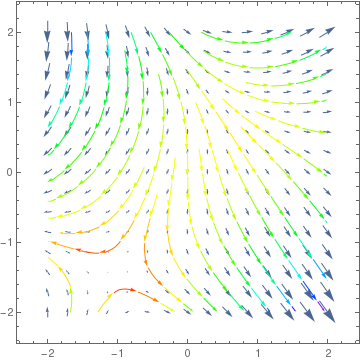We present some codes using Mathematica with different options. VectorDensityPlot[{y, x^2 + y^2*x - 3*y}, {x, -3, 3}, {y, -3, 3}, StreamPoints -> {{{{-2, 1}, Green}, {{1.5, -1}, Red}, Automatic}}][ VectorDensityPlot[{y, x^2 + y^2*x - 3*y}, {x, -3, 3}, {y, -3, 3}, ColorFunction -> "ThermometerColors"][ VectorPlot[{y, x^2 + y^2*x - 3*y}, {x, -3, 3}, {y, -3, 3}, VectorStyle -> {Black, "Disk"}]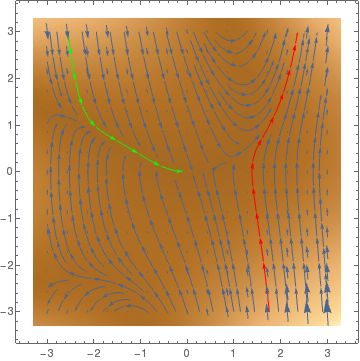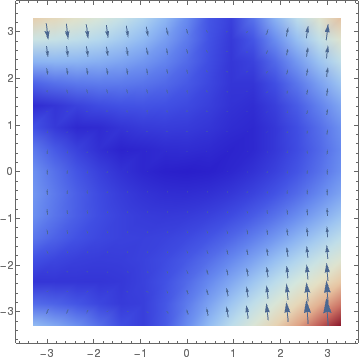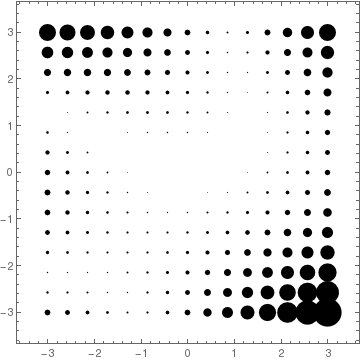StreamPlot[{y, x^2 + y^2*x - 3*y}, {x, -3, 3}, {y, -3, 3}, StreamPoints -> {{{{-2, 1}, Green}, {{1.5, -1}, Red}}}] StreamPlot[{y, x^2 + y^2*x - 3*y}, {x, -3, 3}, {y, -3, 3}, StreamPoints -> {{{{-2, 1}, Green}, {{1.5, -1}, Red}, Automatic}}] StreamPlot[{y, x^2 + y^2*x - 3*y}, {x, -3, 3}, {y, -3, 3}, StreamPoints -> Coarse] StreamPlot[{y, x^2 + y^2*x - 3*y}, {x, -3, 3}, {y, -3, 3}, StreamPoints -> Fine]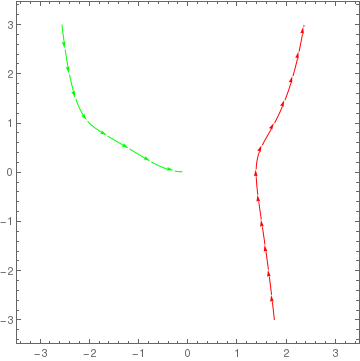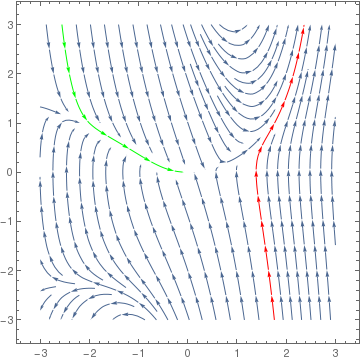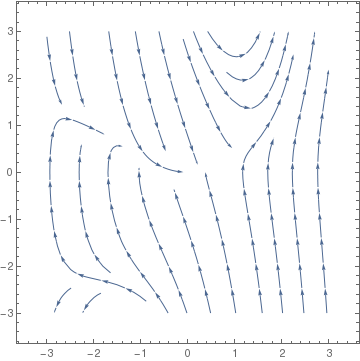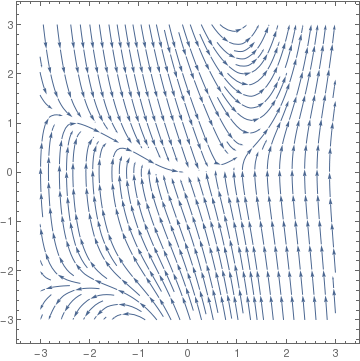ContourPlot[x^2 + y^2*x - 3*y, {x, -3, 3}, {y, -3, 3}, ContourLabels -> All, Contours -> {-2, -1, 0, 1, 2}] ContourPlot[x^2 + y^2*x - 3*y, {x, -3, 3}, {y, -3, 3}, ContourLabels -> All, ColorFunction -> "Rainbow"]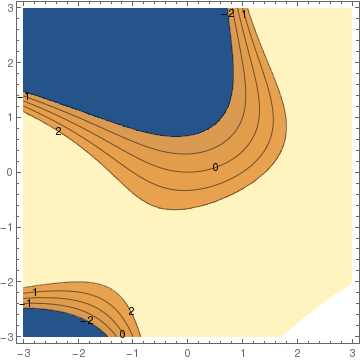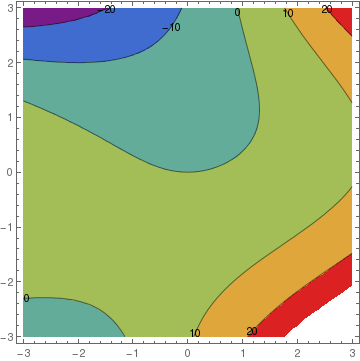Since not every vector field is a gradient field of any function, we need a procedure that calculate it. Finally, here is a subroutine to plot a gradient field based on a (scalar) potential function. gradientFieldPlot[f_, rx_, ry_, opts : OptionsPattern[]] := Module[ { img, cont, densityOptions, contourOptions, frameOptions, gradField, field, fieldL, plotRangeRule, rangeCoords }, densityOptions = Join[ FilterRules[{opts}, FilterRules[Options[DensityPlot], Except[{Prolog, Epilog, FrameTicks, PlotLabel, ImagePadding, GridLines, Mesh, AspectRatio, PlotRangePadding, Frame, Axes}]]], {PlotRangePadding -> None, Frame -> None, Axes -> None, AspectRatio -> Automatic} ]; contourOptions = Join[ FilterRules[{opts}, FilterRules[Options[ContourPlot], Except[{Prolog, Epilog, FrameTicks, PlotLabel, Background, ContourShading, PlotRangePadding, Frame, Axes, ExclusionsStyle}]]], {PlotRangePadding -> None, Frame -> None, Axes -> None, ContourShading -> False} ]; gradField = ComplexExpand[{D[f, rx[]], D[f, ry[]]}]; fieldL = DensityPlot[Norm[gradField], rx, ry, Evaluate@Apply[Sequence, densityOptions]]; field=First@Cases[{fieldL},Graphics[__],\[Infinity]]; img = Rasterize[field, "Image"]; plotRangeRule = FilterRules[Quiet@AbsoluteOptions[field], PlotRange]; cont = If[ MemberQ[{0, None}, (Contours /. FilterRules[{opts}, Contours])], {}, ContourPlot[f, rx, ry, Evaluate@Apply[Sequence, contourOptions]] ]; frameOptions = Join[ FilterRules[{opts}, FilterRules[Options[Graphics], Except[{PlotRangeClipping, PlotRange}]]], {plotRangeRule, Frame -> True, PlotRangeClipping -> True} ]; rangeCoords = Transpose[PlotRange /. plotRangeRule]; If[Head[fieldL]===Legended,Legended[#,fieldL[]],#]&@ Apply[Show[ Graphics[ { Inset[ Show[SetAlphaChannel[img, "ShadingOpacity" /. {opts} /. {"ShadingOpacity" -> 1}], AspectRatio -> Full], rangeCoords[], {0, 0}, rangeCoords[] - rangeCoords[]] }], cont, StreamPlot[gradField, rx, ry, Evaluate@FilterRules[{opts}, StreamStyle], Evaluate@FilterRules[{opts}, StreamColorFunction], Evaluate@FilterRules[{opts}, StreamColorFunctionScaling], Evaluate@FilterRules[{opts}, StreamPoints], Evaluate@FilterRules[{opts}, StreamScale]], ## ] &, frameOptions] ] Here is an example of how to use the function. The contour lines of the potential are shown in white, and the streamlines of the gradient field are orange. gradientFieldPlot[(y^2 + (x - 2)^2)^(-1/ 2) - (y^2 + (x - 1/2)^2)^(-1/2)/2, {x, -1.5, 3.5}, {y, -1.5, 1.5}, PlotPoints -> 50, ColorFunction -> "BlueGreenYellow", Contours -> 16, ContourStyle -> White, Frame -> True, FrameLabel -> {"x", "y"}, ClippingStyle -> Automatic, StreamStyle -> Orange, ImageSize -> 500, GridLinesStyle -> Directive[Thick, Black], "ShadingOpacity" -> .8, Axes -> {True, False}, AxesStyle -> Directive[Thickness[.03], Black], Method -> {"AxesInFront" -> False}]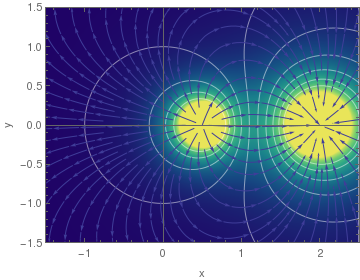How to recognize a gradient system A system of autonomous differential equations \begin{equation} \label{EqGrad.2} \dot{\bf x} = f({\bf x}) \end{equation} is a gradient system if and only if there is a scalar valued function G so that \[ f({\bf x}) = \nabla \cdot G = \left( \frac{\partial G}{\partial x_1} , \frac{\partial G}{\partial x_2} , \ldots , \frac{\partial G}{\partial x_n} \right) .$
In one dimensional case, a gradient system coinsides with an autonomous equation
$x' = f(x) = \frac{{\text d}G(x)}{{\text d} x} .$
In a two-dimensional case, a system
$\dot{x} = f(x,y) , \qquad \dot{y} = g(x,y)$
is a gradient sytem if and only if there is a function G such that
$\frac{\partial G}{\partial x} = -f(x,y) , \qquad \frac{\partial G}{\partial y} = -g(x,y)$
A necessary and sufficent condition for the system to be gradient is the equality of mixed partials,
$\frac{\partial f}{\partial y} = \frac{\partial g}{\partial x} .$
In the general case, the necessary and sufficient condition is again equality of mixed partials expressed as
$\frac{\partial f_i}{\partial x_j} = \frac{\partial f_j}{\partial x_i} ,\quad i,j = 1,2,\ldots , n.$

Equilibria and their stability

Let V be a C¹ function defined on an open neighborhood U1U ⊂ ℝn of the equilibria x*U1. Let us define the associated function via differential equation
$\dot{V} ({\bf x}) = - \sum_{i=1}^n \frac{\partial G}{\partial x_i} ({\bf x})\,\frac{\partial V}{\partial x_i} ({\bf x}) , \qquad {\bf x} \in U_1 .$
If x(t) is a solution of Eq.\eqref{EqGrad.1}, it follows from the chain rule that
\begin{equation} \label{EqGrad.3} \dot{V} ({\bf x}) = \frac{\partial \left( V \circ {\bf x} \right)}{\partial t} (t) . \end{equation}
Theorem 1: The function V is a Liapunov function for the system $$\dot{\bf x} = - \nabla \cdot V({\bf x}) .$$ Moreover, $$\dot{V}({\bf x}) = 0$$ if and only if x is an equilibrium point.
The study of gradient systems \eqref{EqGrad.1} is particularly simple due to the formula
\begin{equation} \label{EqGrad.4} \frac{\partial \left( G \circ {\bf x} \right)}{\partial t} (t) = \nabla G({\bf x}) \cdot \dot{\bf x}(t) = -k \left\vert \nabla G({\bf x}(t)) \right\vert^2 \le 0, \end{equation}
where x(t) is a solution of Eq.\eqref{EqGrad.1}.
Theorem 2: An equilibrium point x*U of the system \eqref{EqGrad.1} is asymptotically stable if and only if x* is an isolated critical point of the function G at which G attains a local minimum.
To understand a gradient flow geometrically we look at thelevel surfacesof the function G :  ℝn → ℝ. These are the subsets $$G^{-1} (c)$$ with c ∈ ℝ. Solutions of the gradient vector field cross the level sets of the function G orthogonally except at critical points. Another consequence of Eq.\eqref{EqGrad.4} is that a gradient system cannot have any periodic solutions.
Theorem 3: Let $$\displaystyle H({\bf x}) = \left[ \frac{\partial^2 G({\bf x})}{\partial x_i \partial x_j} \right]$$ be the Hesian matrix for the gradient system $$\displaystyle \dot{\bf x} = - \nabla \cdot G .$$
• If the eigenvalues of the Hessian are all strictly positive, then the critical point is asymptotically stable.
• If the Hessian has a negative real eigenvalue, then the equilibrium is unstable.

Example: This seems to have Let

$G(x,y) = y^2 (y-1)^2 + 3 x^2$
be the function G :  ℝ² → ℝ. Then the corresponding gradient system is given by
$\begin{split} \dot{x} &= - \partial G/\partial x = -6x, \\ \dot{y} &= - \partial G/\partial y = -2y\left( y-1 \right)^2 - 2y^2 \left( y-1 \right) = -2y \left( y-1 \right)\left( 2y-1 \right) . \end{split}$
The system has three equilibrium points: $O(0,0), \qquad A(0,1/2), \qquad B(0,1)$ The Hessian for the given system is
$H(x,y) = \begin{bmatrix} 6 & 0 \\ 0&2\left( 6y^2 -6y+1 \right) \end{bmatrix} .$
At these three critical points, the Hessian becomes
$H_0 = \begin{bmatrix} 6 & 0 \\ 0&2 \end{bmatrix} , \qquad H_A = \begin{bmatrix} 6 & 0 \\ 0&-1 \end{bmatrix} , \qquad H_B = \begin{bmatrix} 6 & 0 \\ 0&2 \end{bmatrix} .$
Hence, (0,0) and B(0,1) are sink points, but another critical point A(0,1/2) is a saddle point.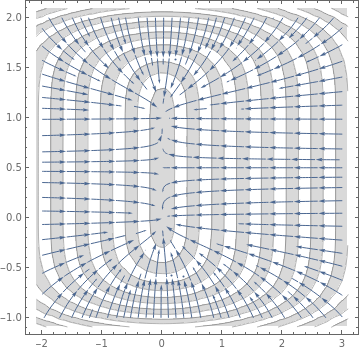StreamPlot[{-6*x, -2*y*(y - 1)*(2*y - 1)}, {x, -2, 3}, {y, -1, 2}, Mesh -> 18, MeshShading -> {LightGray, None}, PlotTheme -> "Detailed"] Figure 1: Gradient system. Mathematica code

■
There is a related concept of the gradient field, where you look at the gradient (vector derivative) of a function:
f[x_, y_] := Sin[x y^2]
D[f[x, y], {{x, y}}]
VectorPlot[%, {x, -1, 1}, {y, -1, 1}]
Out= {y^2 Cos[x y^2], 2 x y Cos[x y^2]}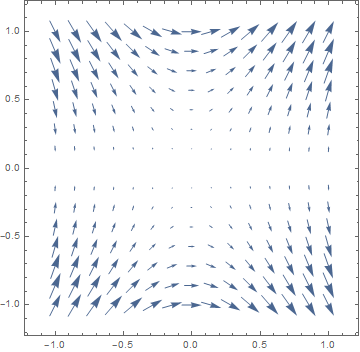1. Calle Ysern, B., Asymptotically stable equilibria of gradient systems, American Mathematical Monthly, 2019, Vol. 126, No. 10, pp. 936--939.
2. Hirsch, M.W., Smale, S., and Devaney, R.L., Differential Equations, Dynamical Systems, and an Introduction to Chaos, 2003, Second edition, Academic Press.